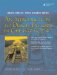# Pointer Pathology

In Section 1.12 we introduced pointers and demonstrated some of the basics of working with them. We now look at two short code examples to demonstrate some of the weird and dangerous things that can happen when pointers are not handled correctly.

Example 22.1. src/pointers/pathology/pathologydecls1.cpp

 ```[ . . . . ] int main() { int a, b, c; <-- 1 int* d, e, f; <-- 2 int *g, *h; <-- 3 int* i, * j; <-- 4 return 0; } ```   (1)As expected, this line creates three ints. (2)This line creates one pointer to an int and two ints. (!) (3)This line creates two pointers to int. (4)This line also creates two pointers to int.

Example 22.1 shows a few of the many ways one can declare pointers. A beginner would be forgiven for thinking the second line of main() creates three pointersafter all, in line one, similar syntax creates three integers. However, when multiple variables are declared on one line, the * type modifier symbol applies only to the variable that immediately follows it, not the type that precedes it. Since whitespace is ignored by the compiler, the location of whitespace can help or confuse the reader.

Example 22.2. src/pointers/pathology/pathologydecls2.cpp

 ```[ . . . . ] int main() { int myint = 5; int *ptr1 = &myint; cout << "*ptr1 = " << *ptr1 << endl; int anotherint = 6; // *ptr1 = &anotherint; <-- 1 int *ptr2; <-- 2 cout << "*ptr2 = " << *ptr2 << endl; *ptr2 = anotherint; <-- 3 int yetanotherint = 7; int *ptr3; ptr3 = &yetanotherint; <-- 4 cout << "*ptr3 = " << *ptr3 << endl; *ptr1 = *ptr2; <-- 5 cout << "*ptr1 = " << *ptr1 << endl; return 0; } [ . . . . ] ```   (1)errorinvalid conversion from int* to int (2)uninitialized pointer (3)unpredictable results (4)regular assignment (5)dangerous assignment!

Example 22.2 is broken up into three sections. Only the first and third sections are equivalent; the second contains a common beginner's mistake.

```src/pointers/pathology> g++ pathologydecls2.cpp
pathologydecls.cpp: In function 'int main()':
pathologydecls.cpp:17: error: invalid conversion from 'int*' to 'int'
src/pointers/pathology>
```

After commenting out the invalid conversion, we can try again.

```*ptr1 = 5
*ptr2 = 1256764
*ptr3 = 7
*ptr1 = 6
```

The value of *ptr2 is unpredictable.

Dereferencing uninitialized pointers for read purposes is bad enough, but then we wrote to it. This is a form of memory corruption, which can cause problems later in the program's execution. Notice the inconsistent value that *ptr1 obtained from *ptr2.

### Further Pointer Pathology with Heap MemoryAn Introduction to Design Patterns in C++ with Qt 4
ISBN: 0131879057
EAN: 2147483647
Year: 2004
Pages: 268